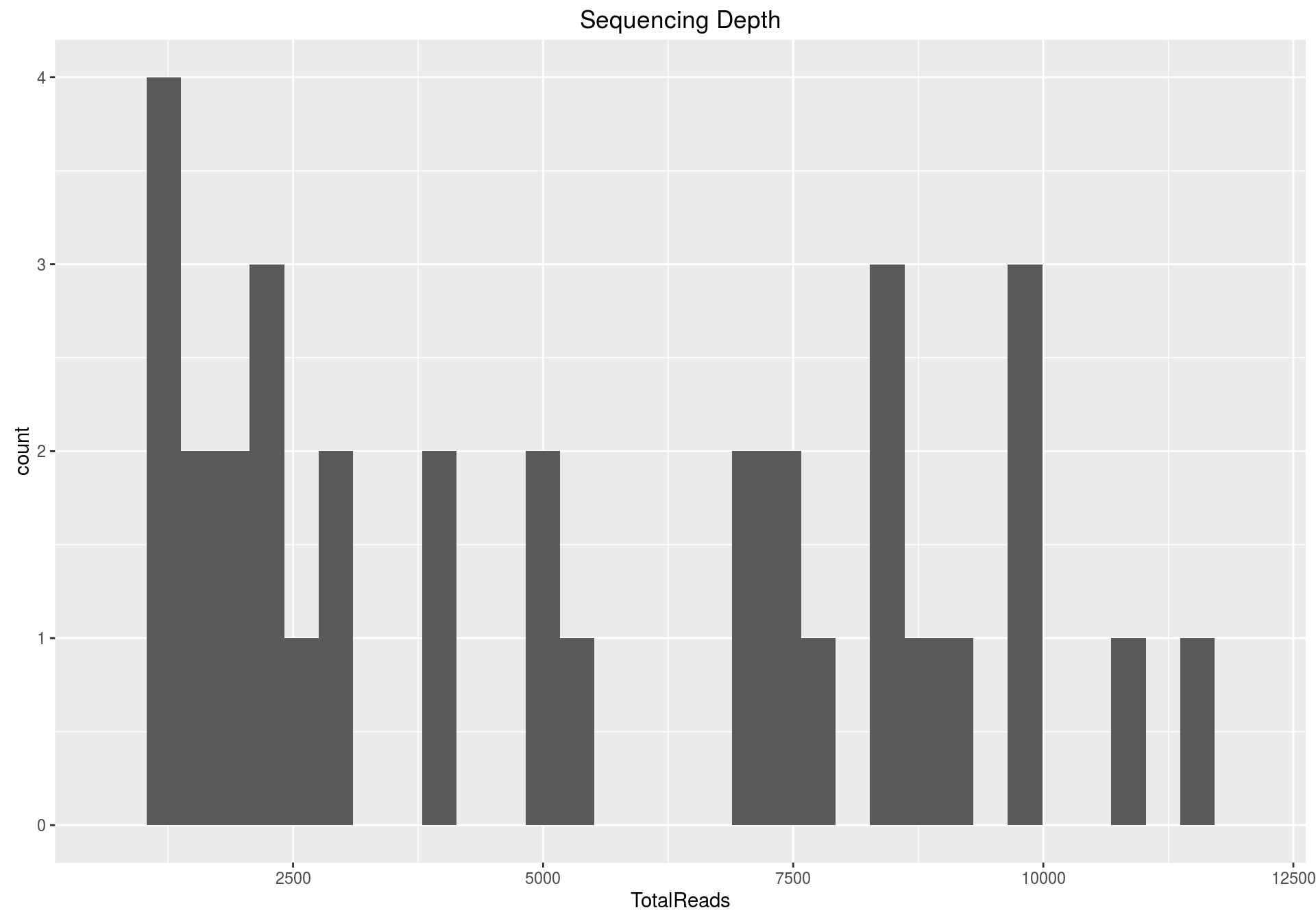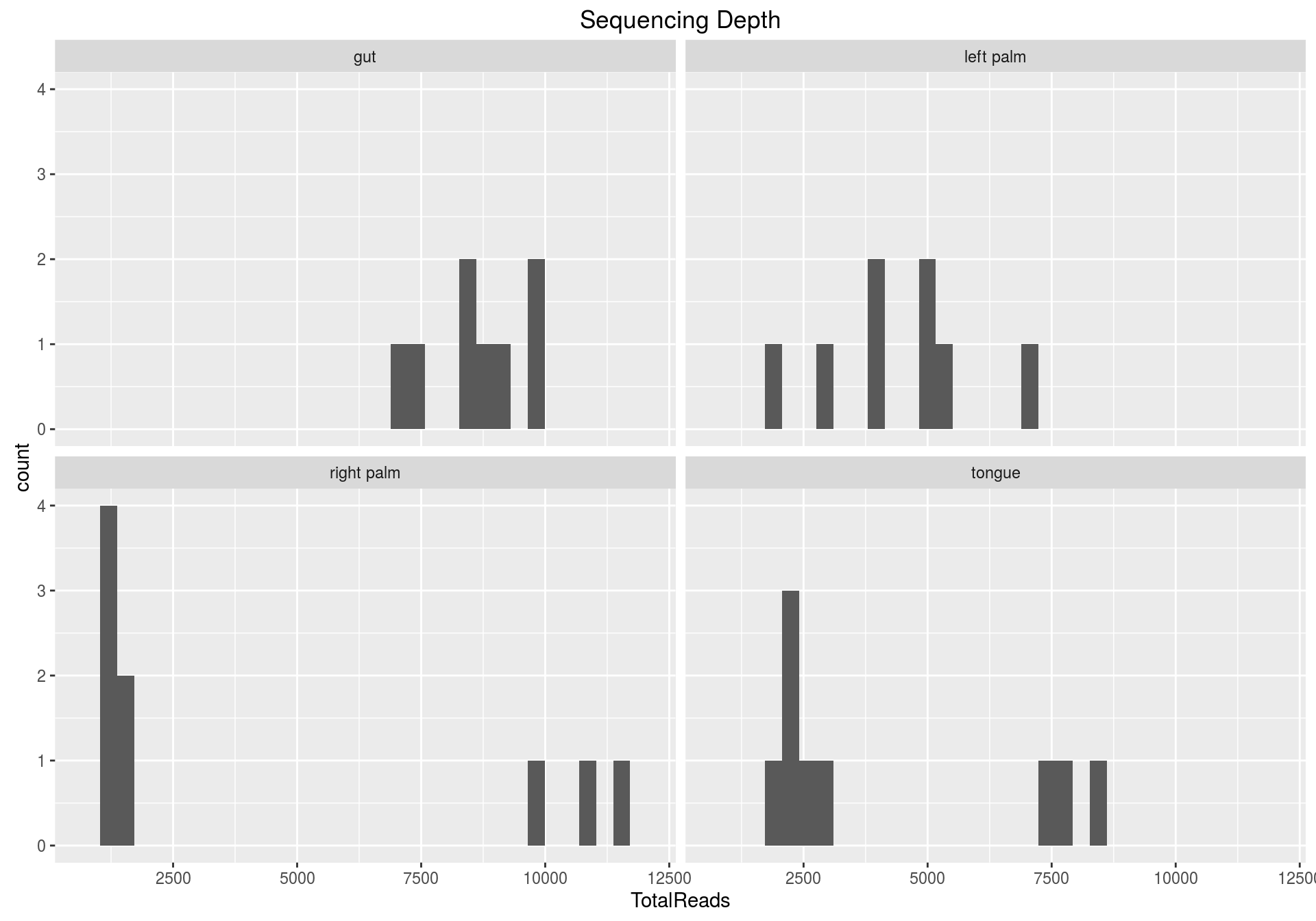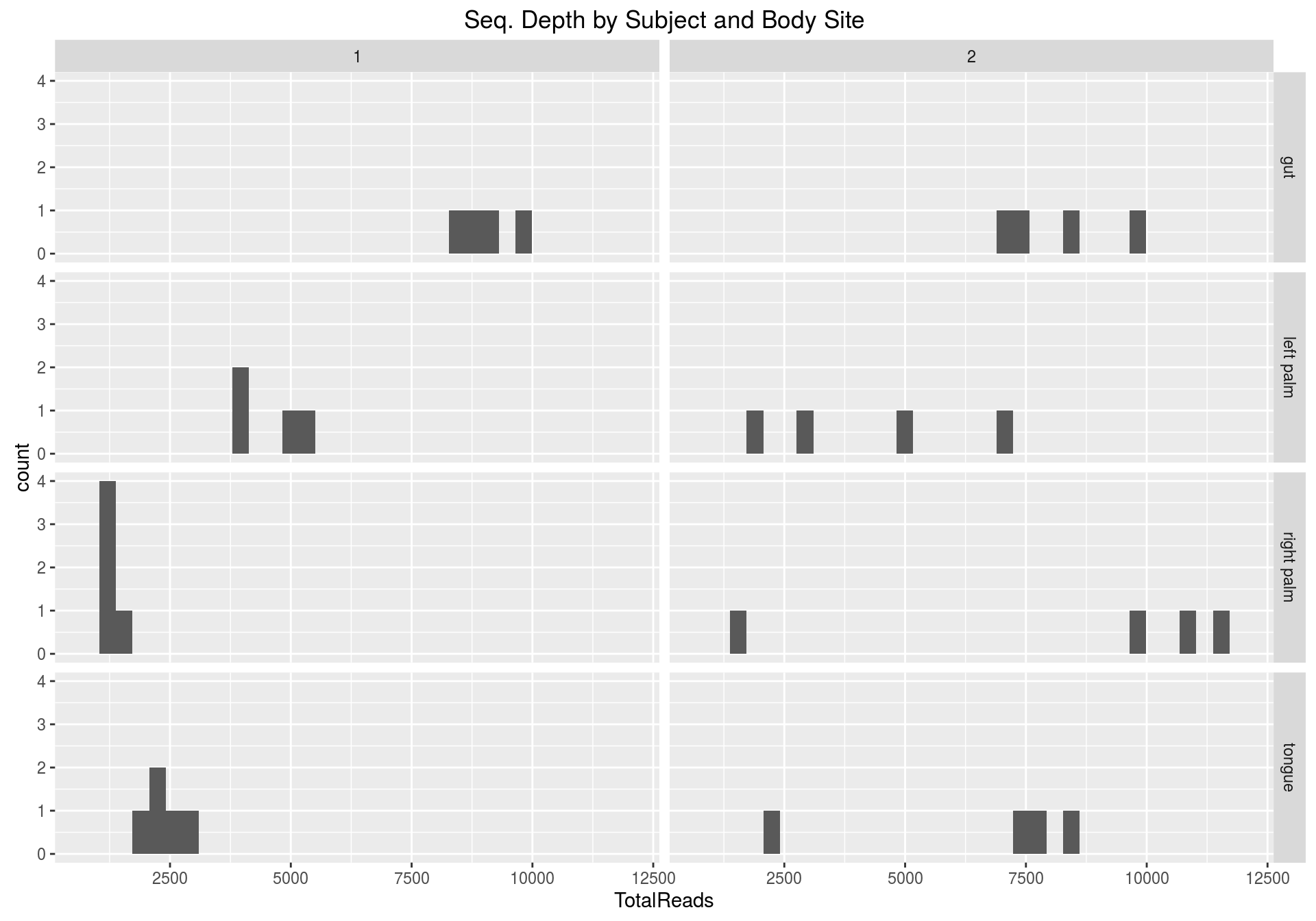There thousands of publicly available R extensions, AKA “packages”. They are not all installed on your system, and only core R packages are loaded when you start a new R session. The `library()` function is used to load non-core packages so that subsequent commands are searched against the functions and data in these packages as well as the core.

I like to also show the package version number in my Rmd workflows (using `packageVersion()`) so that I know what was used at the time the code was executed.

I also like to include a date stamp. There’s one above, but here’s how you can include it in your code:

`gsub("[[:punct:][:space:]]", "-", Sys.time())`

``gsub("[[:punct:][:space:]]", "-", Sys.time())``
``##  "2016-01-18-15-55-00"``

## Load phyloseq and other packages

1. Load the phyloseq, data.table, and ggplot2, packages using the `library()` command.
2. Check the version number of each package using the `packageVersion()` command.
``library("phyloseq"); packageVersion("phyloseq")``
``````## Warning: replacing previous import by 'ggplot2::Position' when loading
## 'phyloseq'``````
``````## Warning: replacing previous import by 'scales::alpha' when loading
## 'phyloseq'``````
``##  '1.14.0'``
``library("data.table"); packageVersion("data.table")``
``##  '1.9.6'``
``library("ggplot2"); packageVersion("ggplot2")``
``##  '2.0.0'``

## Load Pre-Organized Data from Previous Section

In the previous section you organized our Moving Pictures example data using phyloseq tools, and then saved this data from your R session (system memory, RAM) to the hard disk as a compressed, serialized file (extension “`.RDS`”). This is a handy way to store complicated single data objects for later use, without needing to define and document one or more file formats. This data was imported from 3 or 4 QIIME output files in various locations, but now this one self-describing file is all you need.

1. Load your Moving Pictures RDS data file into your R session using the `readRDS()` function, and store the result as an object named `mp`.
``mp = readRDS("mp-phyloseq-lab.RDS")``

Reminder: For storing many objects from an R session at once, try `save()` or `save.image()`, and `load()`.

# Initial exploration of data

Explore the data. Executing a command in the terminal that is just a data object invokes the `print()` or `summary()` functions, which usually give summary details about the data if it is a complex object (like ours), or show the data directly if it is simple. If it is simple but large, you might hit the streaming limits in your console, so try and check sizes first. The “Environment” box in your RStudio console usually tells you these details as well.

1. “print” to screen the `mp` object
2. This will give you other details about the object, as well as a few key functions that can be used to access parts of it. This data is too large to show all in the console, with the exception of `phy_tree()` and `refseq()`, which have their own special print summary functions.

3. Print `mp` to the screen.
4. Get the number of samples and number of taxa (OTUs) directly, using the `nsamples()` and `ntaxa()` functions.
5. The `sample_data` and `tax_table` components of this phyloseq object have their own special variables, namely `sample_variables()` and `rank_names()`. Use these functions on `mp`. What do you get? What does it mean?
6. Find details about the phylogenetic tree in this data using the `phy_tree()` function. What is it telling you? How many leaves are in the tree? How many internal nodes?
7. You could attempt to plot this tree with the default `plot()` function, but it has way too many leaves to be legible. Also, the default plot function is limited, so we’ll come back to this and how to use the phyloseq function `plot_tree()` later on.

``mp``
``````## phyloseq-class experiment-level object
## otu_table()   OTU Table:         [ 3426 taxa and 34 samples ]
## sample_data() Sample Data:       [ 34 samples by 11 sample variables ]
## tax_table()   Taxonomy Table:    [ 3426 taxa by 7 taxonomic ranks ]
## phy_tree()    Phylogenetic Tree: [ 3426 tips and 3424 internal nodes ]``````
``nsamples(mp)``
``##  34``
``ntaxa(mp)``
``##  3426``
``sample_variables(mp)``
``````##   "X.SampleID"               "BarcodeSequence"
##   "Year"                     "Month"
##   "Day"                      "Subject"
##   "ReportedAntibioticUsage"  "DaysSinceExperimentStart"
##  "Description"``````
``rank_names(mp)``
``##  "Kingdom" "Phylum"  "Class"   "Order"   "Family"  "Genus"   "Species"``

## Summarize sequencing depths, in general and by category

Create histograms that summarize sequencing depth in this data. Start with overall, but then also create category-specific histograms to help evaluate the balance in this experiment.

For this we are going to use the ggplot2 package.

1. Define a data.frame or data.table that contains the total number of reads per sample using the `sample_sums()` function, and the `geom_histogram()` geometric object from ggplot2.
2. Separate these histograms by SampleType in separate plot panels using the `facet_wrap()` or `facet_grid()` functions.
3. Add an informative title using the `ggtitle()` function.
4. Modify the axis labels using the `xlab()` and `ylab()` functions.
5. Make a grid of panels by `SampleType` and `Subject` using the `facet_grid()` function. Does it look like there are any imbalances in total counts or number of samples among these critical groups?
``````sdt = data.table(as(sample_data(mp), "data.frame"),
TotalReads = sample_sums(mp), keep.rownames = TRUE)
setnames(sdt, "rn", "SampleID")
pSeqDepth = ggplot(sdt, aes(TotalReads)) + geom_histogram() + ggtitle("Sequencing Depth")
pSeqDepth``````
``## `stat_bin()` using `bins = 30`. Pick better value with `binwidth`.````pSeqDepth + facet_wrap(~SampleType)``
``## `stat_bin()` using `bins = 30`. Pick better value with `binwidth`.````````pSeqDepth +
facet_grid(SampleType ~ Subject) +
ggtitle("Seq. Depth by Subject and Body Site")``````
``## `stat_bin()` using `bins = 30`. Pick better value with `binwidth`.``The lowest number of reads per sample was 1114. That is low for typical new projects, but not that unusual compared to the rest of the samples in this tutorial dataset. From these plots, I don’t recommend filtering any samples based on depth alone, but keep in mind that in some cases you might, mainly when the number of read counts in a sample is so low that it is apparent that something went wrong with that sample. We will keep an eye out for artifacts and odd behavior (outliers) as we go along.

The plots above also do not indicate strong biases in sequencing depth by group or subject. Do you agree?

## Sequencing depth across time

Here we want to check for any associations with sequencing depth and time. This is worthwhile to check early on in an experiment, as metadata variables like sequencing depth should be uncorrelated with key experimental variables. Why is that? What would it mean if there was a strong correlation between depth and variable?

1. Create a ggplot graphic in which the horizontal axis is mapped to `DaysSinceExperimentStart`, the vertical axis is mapped to `TotalReads`, and the color variable is mapped to `Subject`.
2. Make this a scatter plot using the `geom_point()` function.
3. Add a title the same way you did in the previous graphic. Label the graphic Sequencing Depth vs. Time.
4. Scale the vertical axis to base-10 logarithm using the `scale_y_log10()` layer function. Does this improve the plot?
5. What is the minimum number of reads for any sample? (That is, what is the smallest library size?). Does this seem like a problem? Would you remove any samples?

Note that you should enter Subject as `as.factor(Subject)` to let ggplot2 know that this is really a discrete variable, even though it is encoded as a numeric vector of `1` and `2`. Alternatively you could modify the `Subject` variable to be a character or factor, or you could add a new variable that holds `Subject` with a discrete data type; however, I prefer to not modify the original data unless necessary or useful, and the `as.factor()` expression is an elegant option in this case. Why might it be a good idea to avoid modifying key variables in the data?

``````ggplot(sdt, aes(DaysSinceExperimentStart, TotalReads, color = as.factor(Subject))) +
geom_point(size = 5) +
geom_smooth(method = lm) +
ggtitle("Sequencing Depth vs. Time") +
scale_y_log10() +
facet_grid(SampleType ~ .)``````